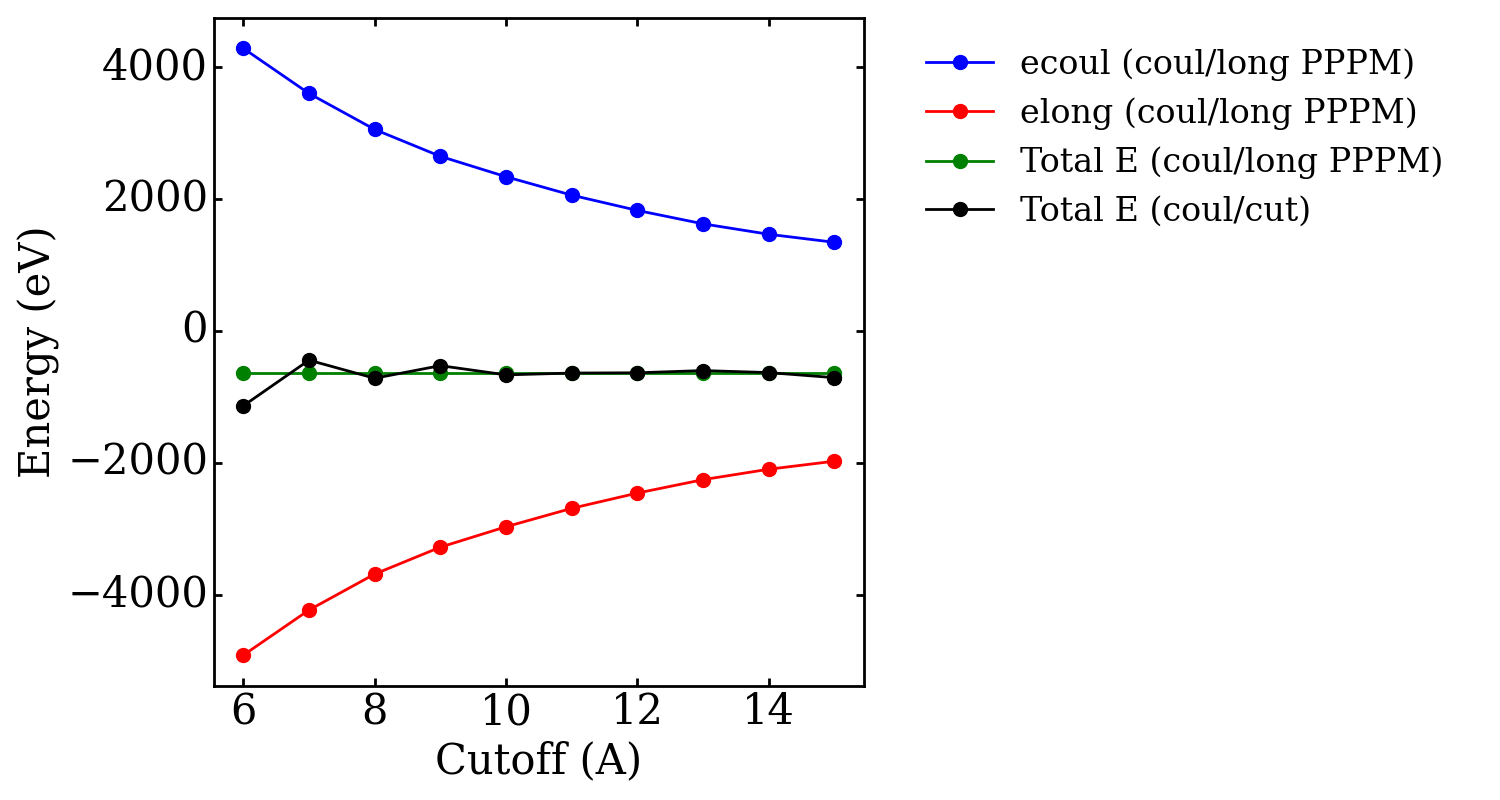# long-range coulombic energy

Hello,

I am trying to determine the pair-wise long-range contribution of the total energy of a system, or the total interaction energy past a certain cutoff. The system I am working with is SPCE water with the following interaction parameters:

pair_style lj/cut/coul/long 12.0 12.0

pair_modify shift yes

kspace_style pppm 1.0e-6

I know with thermo_style, I can print ‘ecoul’ the coulombic energy computed in real space and ‘elong’ the coulombic energy computed in reciprocal space with pppm. However, I have read in other references that the reciprocal space portion of the total coulombic energy spans not only the long-range regime, but also the short range (within defined cutoff). If this is true, then ‘elong’ is not providing just the total interaction energy outside of the cutoff. So I tried the following:

Interaction energy past cutoff of 12 A = [PE using lj/cut/coul/long] - [PE using lj/cut/coul/cut], where both pieces are calculated using cutoff=12 A.

However, even with this method, I am getting a distribution of long-range energies that are comparable or even larger than the total PE. I would have expected the energy contribution from outside of the cutoff to be quite small, so it seems that there is something that I am not understanding. Could you provide some suggestions on what might be wrong with this method for calculating energy outside the cutoff? Or other ideas to calculate this in Lammps?

Thanks for the help in advance!

Jo

You first need to look into understanding how lattice sums like ewald summation work (and corresponding grid based methods like PPPM). You are apparently misguided by lacking sufficient understanding of this method.

The bottom line is: the total coulomb energy is the sum of ecoul and elong. always. how much of that total coulomb interaction is contributed by real space and how much by reciprocal space depends on the width parameter “alpha” to the “damping function” that gets added in real space and subtracted in reciprocal space to generate two well converging sums.
this alpha parameter and the reciprocal cutoff are usually automatically determined from the given coulomb cutoff and the requested accuracy parameter of the kspace style.

you can verify this by setting up a simple test system and compare computing coulomb interactions (only) with run 0 for different choices of the cutoff and the same accuracy requirement for kspace and then also do a test to see the limit for ecoul only when using a coul/cut pair style for the limit of large cutoffs. it should approach the value of the sum of ecoul and elong.

please also note that the reciprocal space contribution is a “manybody” contribution denoting the interaction of the individual charges with all other charges with infinite replication.

axel.

Hi Axel,

Thank you for the feedback! I did the test you mentioned with only intermolecular coulombic interactions and the result is as you described - coul/cut with longer cutoffs will approach ecoul+elong when a long range solver is used. While the damping function is useful to achieve proper convergence, I am still not sure how to isolate E(r>cutoff) the energy that is past the real space cutoff when the damping function is changing based on multiple parameters - cutoff and alpha, or cutoff and accuracy. I see that kspace_modify can be used to control each parameter separately but it is not recommended. I can do further testing on these parameters, but I am not sure tuning these parameters will help me achieve just the energy that is past the cutoff. Could you provide suggestions on how to isolate the proportion of the total interaction energy that is past the cutoff?
Thanks!First you need to explain what it is exactly that you want to determine and what you need it for and why,

As I already explained, the sum of ecoul and elong is the full coulomb interaction energy to an infinitely large cutoff. A partitioning of the long-range term is impossible because it contains contributions from an infinite number of periodic replicas. With optimal parameters, there should not be any (significant) contribution to the real-space part beyond the cutoff.

Axel.